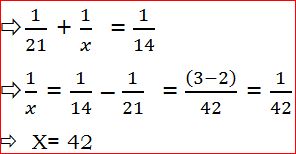# Time & Work - Tricks With Examples## INTRODUCTION:-

Work to be done is generally considered as one unit. It may be constructing a wall or laying a road, filling up or emptying a tank or cistern or eating certain amount of food. If there is more than one person (or thing) carrying out the work, it is assumed that each person (or thing) does the same amount of work each day & all the persons ( or things) do exactly the same amount of work.

### For Example:-

• If a person completes a work in 4 days, he does (¼ )th of the work each day & conversely, if a person can complete (¼)th of a work in one day, he can complete the work in 4 days.
• If a tap can fill a tank in 20 minutes, then in one minute, it can fill (1/20)th part of the tank.
• If 2 people together can do the work in 8 days, it means that one man can do it in 16 days. This in turn, means each person can do (1/16)th of the work per day.
• If a girl works three times as fast as a boy, the girl takes one-third of the time the boy takes to complete the work. If the boy takes 12 days to complete the work, then the girl takes 4 days to complete the work.
•  In pipes & cisterns, a filling pipe or a tap does positive work & an emptying pipe or a “leak” does negative work.
• Wages earned by people doing a work together are to be distributed in the ratio of the total work done by each of them. If a group of people, working at a different pace (i.e., the work done by each of them per day is different). Start & complete a work together, then the wages or earnings have to be divided in the ratio of the work per day of each of them.

## Examples With Explanation

1) Anil can do a work in 18 days while Bhaskar can do the same work in 36 days. In how many days can both together complete the work?

Solution:-
➡Part of the work done by Anil in one day = 1/18
➡Part of the work done by Bhaskar in one day = 1/36
➡Part of the work done by both together in one day = 1/18+1/36➡Number of days taken by both together to complete the work = 12 days.
Short cut:-➡Anil’s 1 day’s work = 2

➡Bhaskar’s 1 day’s work = 1

➡Anil & Bhaskar’s 1 day’s work = 3

➡Together, both can complete the given work in = 36/3 = 12 days.

2) Raju can complete a work in 21 days. Raju & Pavan together can complete the work in 14 days. In how many days Pavan alone can complete the work?

Solution:-

➡Part of the work done by Raju in one day = 1/21
➡Part of the work done by Raju & Pavan together in one day = 1/14
➡Let Pavan alone complete the work in ‘x’ days.➡Therefore, Pavan takes 42 days to complete the work alone.

#### Short cut:-

 LCM- 42 Raju- 21 days 2 Raju + Pavan – 14 days 3 Pavan- ‘x’ days 3-2=1
➡Pavan alone can complete the given work in = 42/1 = 42 days